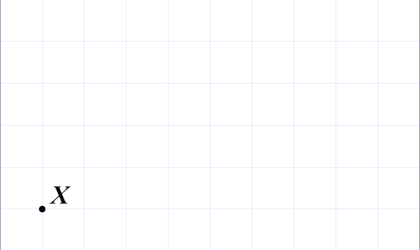# Exam-Style Questions.

## Problems adapted from questions set for previous Mathematics exams.

### 1.

GCSE Higher

(a) Without using a calculator, show that $$\sqrt{28}=2\sqrt7$$

(b) The point $$X$$ is shown on the unit grid below. The point $$Y$$ is $$\sqrt17$$ units from $$X$$ and lies on the intersection of two grid lines. Mark one possible position for $$Y$$.### 2.

GCSE Higher

(a) Express $$\sqrt{5} + \sqrt{20}$$ in the form $$a \sqrt{5}$$ where $$a$$ is an integer.

(a) Express $$( \frac{1}{ \sqrt{5} } ) ^ 9$$ in the form $$\frac{ \sqrt{b}}{c}$$ where $$b$$ and $$c$$ are integers.

### 3.

GCSE Higher

(a) Write this list of numbers in order, smallest first.

$$\sqrt{47}, \frac{22}{3}, 3.5^2, 8.64$$

(b) Write $$(1 + \sqrt5)^2$$ in the form $$a + b\sqrt5$$ .

If you would like space on the right of the question to write out the solution try this Thinning Feature. It will collapse the text into the left half of your screen but large diagrams will remain unchanged.

The exam-style questions appearing on this site are based on those set in previous examinations (or sample assessment papers for future examinations) by the major examination boards. The wording, diagrams and figures used in these questions have been changed from the originals so that students can have fresh, relevant problem solving practice even if they have previously worked through the related exam paper.

The solutions to the questions on this website are only available to those who have a Transum Subscription.

Exam-Style Questions Main Page

Search for exam-style questions containing a particular word or phrase:

To search the entire Transum website use the search box in the grey area below.